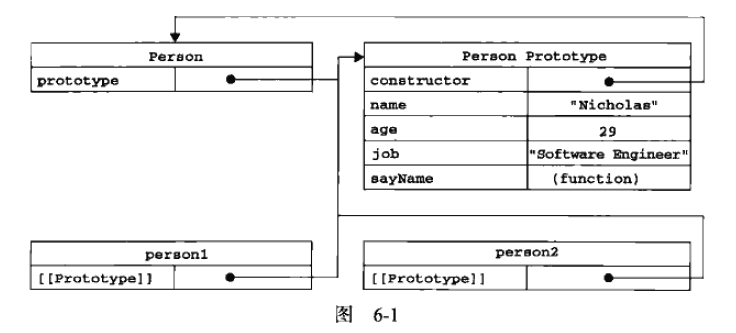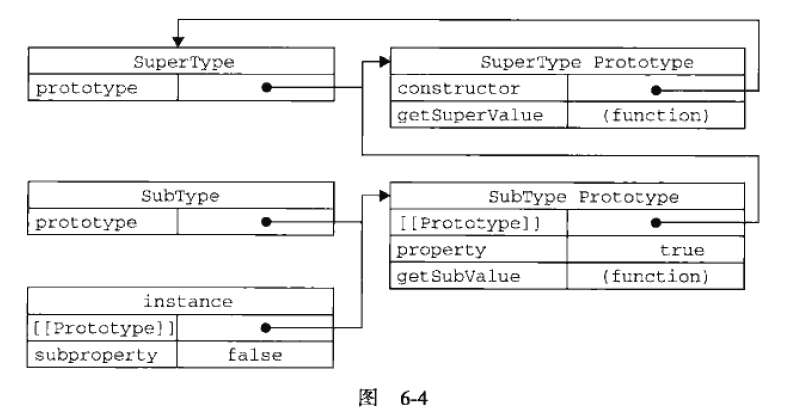# 对象及属性

JS中的对象并不像Python一样通过类来操作，所以在JS内一般不采用类的概念。JS的对象指的是无序属性的集合，类似于一个散列表，就是一个键值对，其中值可以是数据或者函数。

## 对象的属性

### 数据属性

1. [[Configurable]]，是否能通过delete删除该属性，是否能修改属性的特性，是否能将数据属性修改为访问器属性。直接在对象上定义的属性默认该项为true。
2. [[Enumerable]]，是否能通过for-in返回该属性，即对对象调用for-in时，该属性是否包含在返回的内容里。直接在对象上定义的属性默认为true。
3. [[Writable]]，是否能修改属性的值。直接在对象上定义的属性默认为true。
4. [[Value]]，保存了这个属性的数据值，读写的时候都从Value的位置进行读写，这个特性默认为undefined。

```person = {name:"jenny",age:16};

Object.defineProperty(person,"name",{
writable:false,
value:"cony",
});

console.log(person.name);
person.name = "jenny";
console.log(person.name);
```

### 访问器属性

1. [[Configurable]] 与数据属性相同
2. [[Enumerable]] 与数据属性相同
3. [[Get]] 在读取的时候调用的函数，默认为undefined。
4. [[Set]] 在写入的时候调用的函数，默认为undefined。

```let book = {
_year:2004,
edition:2,
};
Object.defineProperty(book,"year",{
get:function () {
return this._year;
},
set:function (newValue) {
if(newValue>2004){
this._year = newValue;
this.edition = 2 + (newValue-2004);
}
else {
this._year = 2004;
this.edition = 2;
}
}
})```

## 对象是什么：建立对象的过程

### 工厂模式

```function createPerson(name,age,job){
var o = new Object();
o.name = name;
o.age= age;
o.job= job;
o.sayName = function () {
console.log(this.name);
};
return o;
}
```

### 构造函数模式

```function Person(name,age,job) {
this.name = name;
this.age=age;
this.job= job;
this.sayName= function () {
console.log(this.name);
};
}
let p1 = new Person("jenny",33,"artist");
let p2 = new Person("cony",4,"magician");
```

### 原型模式

```function Person() {
Person.prototype.name = "jenny";
Person.prototype.age = 33;
Person.prototype.job = "artist";
Person.prototype.sayName = function () {
console.log(this.name)
}
}
let p1 = new Person();
```### 组合使用构造函数和原型模式

```function Person(name,age,job) {
this.name = name;
this.age= age;
this.job = job;
this.friends = ['Minko','cony','arashi']
}

Person.prototype = {
constructor: Person,
sayName: function () {
console.log(this.name)
}
};

let p1 = new Person("jenny",33,'artist');
let p2 = new Person("cony",4,'magician');
```

## 继承

### 基础原型链模式

```function SuperType() {
this.property= true;
SuperType.prototype.getSuperValue = function () {
return this.property;
}
}

function SubType() {
this.subproperty = false;
}

SubType.prototype = new SuperType();

SubType.prototype.getSubValue = function () {
return this.subproperty;
};

let instance = new SubType();
```SubType的原型就是SuperType的实例，SuperType的实例指向SuperType的原型。其实JS内所有的对象都继承Object，所以其实SuperType的原型里还有一个[[prototype]]指针指向Ojbect的原型。

### 借用构造函数模式

```function SuperType() {
this.colors = ['red','blue','green'];
}

function SubType() {
SuperType.call(this);
}

SubType.prototype = new SuperType();

SubType.prototype.getcolors = function () {
console.log(this.colors);
};

let i1 = new SubType();
let i2 = new SubType();
i1.colors.push("white");
i1.getcolors();
i2.getcolors();
```

```function SuperType(name) {
this.name = name;
}

function SubType() {
SuperType.call(this,name);
this.age= age;
}
```

### 组合继承模式

```function SuperType(name) {
this.name = name;
this.colors = ['red','green','blue'];
// 在父类原型上定义方法，也可以被继承到子类
SuperType.prototype.sayName = function () {
console.log(this.name)
}
}

function SubType(name,age) {
SuperType.call(this,name);
this.age= age;
}

// 连接原型链
SubType.prototype = new SuperType();
// 由于调用了父类的构造函数，实际是自己的构造函数发挥作用，所以修改原型的constructor指针
SubType.prototype.constructor = SubType;
// 在子类原型=父类实例上定义方法，可以被所有的子类共享
SubType.prototype.sayAge = function () {
console.log(this.age);
};
let p1 = new SubType('jenny',33);
let p2 = new SubType("cony",4);
```

### 寄生组合式继承模式

```function inheritPrototype(SubType, SuperType) {
let prototype = Object(SuperType.prototype);
console.log(prototype);
prototype.constructor = SubType;
SubType.prototype = prototype;
}

function SuperType(name) {
this.name = name;
this.colors = ['red','green','blue'];
}

SuperType.prototype.sayName = function(){
console.log(this.name);
};

function SubType(name,age){
SuperType.call(this,name);
this.age = age;
}

// 这一行用来替代再次执行父类型构造函数
inheritPrototype(SubType,SuperType);
// SubType.prototype = new SuperType();

SubType.prototype.sayAge = function(){
console.log(this.age)
};

let p1 = new SubType('jenny',33);
let p2 = new SubType("cony",4);
```